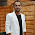## Pages

The blog has moved to the new site F10Debug.com

## Codility binary gap solution C#

Find longest sequence of zeros in binary representation of an integer.
Solution:
public int GetBinaryGap(int N)
{
string binaryValue = Convert.ToString(N, 2);

int longestBinaryGap = 0;
int binaryGapLenght = 0;
for (int i = 1; i < binaryValue.Length; i++)
{
if (binaryValue[i - 1] == '1' && binaryValue[i] == '0')
{
binaryGapLenght = 1;
}
else if (binaryValue[i - 1] == '0' && binaryValue[i] == '0')
{
binaryGapLenght++;
}
else if (binaryValue[i - 1] == '0' && binaryValue[i] == '1')
{
longestBinaryGap = Math.Max(longestBinaryGap, binaryGapLenght);
}
}

return longestBinaryGap;
}

By submitting this answer to codility I got 100% Marks.

#### 3 comments:

1.Not sure how but this looks to be incorrect.

public static int GetLongestSequence(int N)
{
string binary = Convert.ToString(N, 2);
Console.WriteLine("Method 2");
int longest = 0,current = 0;
for (int i = 0; i < binary.Length; i++)
{
if (binary[i] == '0')
{
if (longest == current) longest++;
current++;
}
else
{
current = 0;
}
}

return longest;
}

1.@Ravi mittal - I have tested this in codility and got 100%.

2.It is correct if you ignore the last sequence of 0s, which wasn't mentioned in your post.. I checked the full question on Codality and it is the correct solution.